# Enumerative and Analytic Combinatorics

### Friday January 22

10:00-10:30
Christian Krattenthaler (University of Vienna)
The poset of bipartitions

Bipartitional relations were introduced by Foata and Zeilberger in their characterization of relations which give rise to equidistribution of the associated inversion statistic and major index. I shall consider the natural partial order on bipartitional relations given by inclusion. This partial order gives in fact rise to a lattice which has the remarkable property that the Möbius function of any interval is 0, 1, or -1. However, to prove the latter fact, is surprisingly difficult to prove. It requires Robin Forman's Discrete Morse Theory, the main ideas of which I shall outline. This is joint work with Gabor Hetyei.

11:00-11:30
Josef Cibulka (Charles University, Prague)
Families of permutations with bounded VC-dimension have almost exponential size

A familyof n-permutations has VC-dimension k if k is the largest number such that the elements ofinduce all k! permutations on some k-tuple of indices. An example of a family with VC-dimension k are permutations avoiding some fixed (k+1)-permutation. Marcus and Tardos proved the Stanley-Wilf conjecture, which says that the number of n-permutations avoiding an arbitrary given permutation grows only exponentially in n. Raz showed that if a family of n-permutations has VC-dimension 2, then its size is at most exponential in n.

In contrary to these two results, we find a family of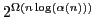permutations with VC-dimension 3. On the other hand, we show the upper bound offor any family of permutations with constant VC-dimension.

We also study a related problem of the maximum number of 1-entries in an n x n (0,1)-matrix with no k-tuple of columns containing all k-permutation matrices. It is known that this number is linear in n if. For any fixed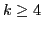, we show bounds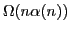and.

This is a joint work with Jan Kynčl.

11:30-12:00
Johannes Morgenbesser (Vienna University of Technology)
Generalized Thue-Morse Sequences of Squares

Let (tn) = (0110100110010110...) be the Thue-Morse sequence. Then the letters 0 and 1 are equally likely. Interestingly, this property persists for subsequences like linear progressions (tan+b). Recently, Mauduit and Rivat could settle the long standing conjecture (attributed to Gelfond) that (tp), p prime, and (tn2) have the same property.

In our work, we consider compact group generalizations T(n) of the Thue-Morse sequence. We prove that the subsequence T(n2) is uniformly distributed with respect to a proper measure, that is absolutely continuous with respect to the Haar measure. The proof is based on a generalization of the Fourier based method of Mauduit and Rivat to group representations. As an application, we show a result on the frequency of letters of subsequences of special automatic sequences.

12:00-12:30
Martin Zeiner (TU Graz)
Convergence properties of q-binomial distributions

We investigate in two q-analogues of the binomial distribution which are connected with basic hypergeometric series (or q-series). We study Kemp's q-binomial distribution (joint work with Stefan Gerhold) and the q-deformed binomial distribution which have applications in biology and physics. Several convergence results involving the classical binomial, the Heine, the Euler, the discrete normal, the Poisson, and the exponential distribution are established. Some of them are q-analogues of classical convergence properties (e.g. there are q-analogues of the convergence of the classical binomial distribution with constant mean to the Poisson distribution or of the binomial distribution to the normal distribution).

14:00-14:30
Stephan Wagner (Stellenbosch University)
Parameters of integer partitions - a zoo of limit laws

Limit laws for various parameters of integer permutations and their generalisations (where the parts are restricted to certain sets, e.g. the set of all squares or all primes) are presented. Various classical distributions (Gaussian, Rayleigh, Gumbel, Geometric) as well as more "exotic" distributions occur as limit laws in this context. For certain parameters, interesting phase transitions can be observed as well.

14:30-15:00
Veronika Kraus (TU Wien)
The degree distribution in Random Unlabelled Subcritical Graph families

We study the distribution of node degrees in subcritical families of graphs. These are families which are block-stable, that is they are determined only by their 2-connected components, and the generating function of 2-connected components fulfills certain analytic conditions, which we call subcriticality conditions. Important subcritical families are outerplanar and series-parallel graphs. We extend recent results by Drmota, Gimenez and Noy in the labelled case, focusing mainly on the unlabelled framework. We use a generating functions approach, taking into account symmetries evolving from unlabelling and therefore using Polya's theory on cycle index sums. By methods of singularity analysis we can prove a central limit law for the number of nodes of given degree k in a random unlabelled graph of size n in any subcritical family of graphs. Therefore we obtain a degree distribution. This results holds generally in the subcritical framework, without any further knowledge on the structure of 2-connected components.

15:00-15:30
Georg Seitz (Vienna University of Technology)
The degree of the nodes in random increasing k-trees

Increasing k-trees are labelled graphs which are recursively defined as follows: The k-clique formed by the nodesis the only increasing k-tree with k nodes. An increasing k-tree with n > k nodes is constructed by connecting a new node n to all nodes of a k-clique in an increasing k-tree with n-1 nodes.

For, we study the probability distribution of the degree of node l+k in random increasing k-trees of size n+k. Using a generating functions approach, we derive the exact distribution for fixed n and l. Furthermore, we fully characterize the limiting behaviour for, both for l fixed and for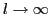. As a corollary, we obtain a weak form of the well-known power law for the degree distribution in increasing k-trees for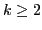.